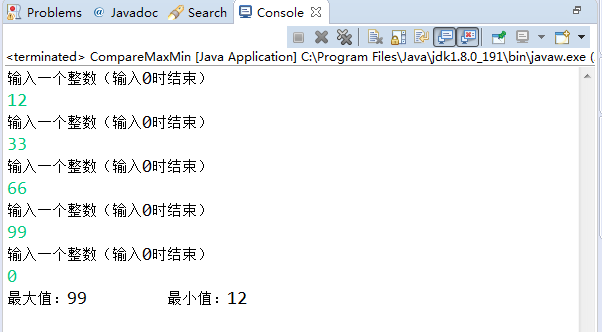• pythonwhile循环while 语句：执行语句结束条件#应用while输出1到11counts = 1while True:print("counts:", counts)counts = counts +1 #counts +=1if counts == 11:breakfor 循环for 变量 in range(起始值...
python
while循环
while 语句：
执行语句
结束条件
#应用while输出1到11
counts = 1
while True:
print("counts:", counts)
counts = counts +1 #counts +=1
if counts == 11:
break
for 循环
for 变量 in range(起始值，终止值，步长):
循环语句
#从零开始以3为步长不超过10
for i in range(0,10,3)
print(i)
python注释中获得输入信息的几种方式
方法1：
变量1 = input（“A”）
变量2 = input（“B”）
info = """
C：%s
D：%s
"""%(变量1，变量2)
#输入姓名、年龄，输出姓名、年龄
name = input("name")
age = int(input("age"))
info = """
-------------info of %s---------
name:%s
age:%d
"""%(name,name,age)
print(info)
方法2：
变量1 = input（“A”）
变量2 = input（“B”）
info = """
C：{E}
D：{F}
""".format(E=变量1，F=变量2)
info2 = """
-------------info of {_name}---------
name:{_name}
age:{_age}
""".format(_name=name,
_age=age)
print(info2)
方法3：
变量1 = input（“A”）
变量2 = input（“B”）
info = """
C：{0}
D：{1}
""".format(变量1，变量2)
info3 = """
-------------info of {0}---------
name:{0}
age:{1}
""".format(name, age)
print(info3)
简单登陆
#引入一个getpass
import getpass
#定义一个用户名和密码
_name = "123"
#获得键盘输入值
name = input("name")
# 密码密文，pycharm不好使
#判断用户名和密码是否一致
#将用户名传给输出语句中
print("----------------")
else:
猜大小小程序
number = 20
#for 循环 range(开始，结束，步长)
for i in range(1,10,2):
#获得输入值
guess_number = int(input("guess number:"))
#进行比较
if guess_number == number:
print("yes, you got it.")
elif guess_number > number:
print("think smaller ...")
else:
print("think bigger!")
print("loop",i)
展开全文• while True: s=input(‘请输入&gt;&gt;&gt;’) if s==’quit()’: break ...print(‘程序结束’) j=1 while j&lt;=5: i=1 while i&lt;=8: print(i) if i=...
while True:
s=input('请输入>>>')
if s=='quit()':
break
print('你刚输入的是'，s)
print('程序结束')

j=1
while j<=5:
i=1
while i<=8:
print(i)
if i==4:
break
i+=1
j+=1
执行结果
1
2
3
4
1
2
3
4
1
2
3
4
1
2
3
4
1
2
3
4
练习：任意输入一些正整数，当输入负数时结束输入
当输入完成后，打印你刚输入这些数的和

习题答案
data=0
while True:
x=int(input('请输入数'))
if x<=0:
print(data)
break
data＋＝x
习题　输入一个整数ｎ打印一个宽度为n个字符宽度的正方型

x=int(input())
i=1
alf='*'
while i<=x:
j=1
while j<=x:
if i==1 or i==x:
print(alf,end=' ')

elif i!=1 or i!=x:
if j==1 or j==x:
print(alf,end=' ')
if j!=1 and j!=x:

print(' ',end=' ')
j+=1

print()
i+=1
输出结果
8
练习：求下列多项式的和
１＋１／２＋１／４＋１／８．。。。。。１／２**1000的和
写程序求1-1/3+1/5-1/7+1/9+...........1/(2*n-1)
求n=10000时此公式的和
将上面结果×４打印
用while语句实现打印等腰直角三角型输入的数为直角边

习题答案

x=int(input())
i=1
num=0
while i<=x:
num+=1/2**(i-1)
i+=1
else:
print(num）

x=int(input())
num=0
i=1
while i<=x:
num+=(1/(2*i-1))*((-1)**(i+1))
i+=1
print(num)
print(4*num)

x=int(input())
i=1
while i<=x:

print(i*'* ',end='')

print()
i+=1

展开全文• python中的循环语句，可使用for循环，或者while循环。两者的区别是，for循环要求提前知道要循环的次数，而...当判断条件假false循环结束。 while 语句还有另外两个重要的命令 continue，break 来跳过循环，con
python中的循环语句，可使用for循环，或者while循环。两者的区别是，for循环要求提前知道要循环的次数，而while循环只要满足条件就会循环，直到条件不符合，跳出循环。下面是while循环的详解
其基本形式为：
while 判断条件：

执行语句……


执行语句可以是单个语句或语句块。判断条件可以是任何表达式，任何非零、或非空（null）的值均为true。
当判断条件假false时，循环结束。
while 语句时还有另外两个重要的命令 continue，break 来跳过循环，continue 用于跳过该次循环，break 则是用于退出循环。
此外"判断条件"还可以是个常值，表示循环必定成立，具体用法如下：

# continue 和 break 用法

i = 1

while i < 10:

i += 1

if i%2 > 0:     # 非双数时跳过输出

continue

print i         # 输出双数2、4、6、8、10

i = 1

while 1:            # 循环条件为1必定成立

print i         # 输出1~10

i += 1

if i > 10:     # 当i大于10时跳出循环

break

展开全文java mysql 数据库 switch
• 输入一批整数，比较并输出最大值和最小值，输入0时结束循环。 分析思路： 首先想起使用循环结构来实现，本题我使用do-while循环并且注意定义变量必须定义在do-while的外层循环。 代码： package Homework1208; ...
输入一批整数，比较并输出最大值和最小值，输入0时就结束循环。
分析思路：
首先想起使用循环结构来实现，本题我使用do-while循环并且注意定义变量必须定义在do-while的外层循环。
代码：

package Homework1208;

import java.util.Scanner;
//比较一批数 最大值和最小值
public class CompareMaxMin {
public static void main(String[] args) {
//输入一批整数，比较并输出最大值和最小值
//输入0时就结束循环
//声明变量
int max = -99999;        //最大整数
int min = 99999;        //最小整数
int aInteger ;    //一整数
Scanner input = new Scanner(System.in);
System.out.println("输入一个整数（输入0时结束）");
//从控制台获取————输入的整数
aInteger = input.nextInt();
//把所输入的整数赋值给最大值和最小值
max = aInteger;
min = aInteger;
do{
System.out.println("输入一个整数（输入0时结束）");
//从控制台获取————输入的整数
aInteger = input.nextInt();
if(aInteger>max) {
//把输入的最大整数赋值给NumMax
max = aInteger;
}else if(aInteger<min&&aInteger!=0) {
//把输入的最小整数赋值给NumMin，并且aInteger不为零
min = aInteger;
}
}while(aInteger!=0);//aInteger不为0，继续循环
System.out.println("最大值："+max+"\t最小值："+min);
}
}

运行结果：展开全文• 题目 需要实现的结果展现 思路 做这道题之前首先要考虑使用什么方法，在上图可以看到这题里存在几个关键字： 输入：首先想到Scanner类 整数：基本数据类型int 当为0结束：这是一个循环体（循环结构：whlie for） ...
• ## Python-输入与循环

千次阅读 2019-01-13 21:45:59
你一定纠结了，今天怎么是这么一个题目，无他，《编程导论》书上有两个小标题，分别是输入循环。要知道，这不是一本专门讲python的语法书，而是比较综合的编程书籍，所以叫编程导论。anyway，还是崇拜一下MIT吧，...教程
• import java.util.Scanner; public class Test01 { public static void main(String[] args) { int max;... // 输入的整数 System.out.println("请输入一个整数："); @SuppressWarnin...
• 获取用户输入后，Python将其存储在一个变量中，以方便你使用。（1）获取数值可以用 int()函数（2）求模运算符（%）将两个数相除并返回余数2.while循环简介（1）for循环用于针对集合中的每个元素都一个代码块，而...
• for和while循环语句用法 ...在python中，while循环，只有结果为真，才会进入循环体，结果为假，就结束循环；所以while很容易就进入死循环，九九乘法表两个while（或者for）循环嵌套就实现了 3...
• ## python循环

千次阅读 2017-05-06 17:37:15
• 【问题描述】从键盘输入非0整数，以输入0输入结束标志，求平均值，统计正数负数个数 【输入形式】 每个整数一行。最后一行是0，表示输入结束。 【输出形式】 输出三行。 第一行是平均值。第二行是...
• Python 中的循环语句有 2 种，分别是 while 循环和 for 循环，while 循环和 if 条件分支语句类似，即在条件（表达式）为真的情况下，会执行相应的代码块。...1.break 结束循环 2.continue 跳出本次循环 break和con...学习编程
• #python中，while语句用于循环执行程序，即在某个条件下，循环执行某段程序，以处理需要重复处理的相同任务。 #while是“当型”循环结构。 i=1 while i&lt;=20: print(i,end=" ") i+=1 sum=0 i=1 ...
• 获取用户输入后，Python将其存储在一个变量中，以方便使用。prompt="if you tell us who you are , we can personlize the message you see." prompt+="\nWhat is your first name?" name=input(pwhile
• "请输入一个整数(输入0结束)：" ); int num= input.nextInt(); int max=num; // 给最大值赋一个初始值 int min=num; // 给最小值赋一个初始值 while (num!=0 ){ System.out.print( "请输入一个整数...
• /usr/bin/python3 i =0 while True: sex = input("Please input your sex (boy or girl):") if sex =="boy": print("你好啊!靓仔") break elif sex == "girl": print("你好啊！靓女") break else: if i<...
• Python-入门中的入门——打印日历题干大意代码语法易错点整理输入输出格式for循环的range 历程： 大一上半年C++书中的案例，连注释带上代码一共二百行，当时自己C++写了足足200行才解决。后来19年元旦自学python拿起...打印日历
• 输入一批整数，输出其中的最大值和最小值，输入数字0结束循环。程序运行结果如图所示 代码 public class Practice2 { public static void main(String[] args) { Scanner input =new Scanner(System.in); int...java 基础
• Python中的用户输入和while循环 1.input()函数的工作原理 程序在运行到input函数的时候回暂停，然后输出input函数括号中的提示参数，等待用户输入一些文本。再用户输入完后再讲该文本存储在一个变量中。 使用方法： ...
• 本文介绍python中if...else..条件判断以及while...循环和for...的语法格式和使用方式，属于python入门基础。if判断
• 不确定python中for循环每次的结束位置。和C语言不一样，python的for循环没有括号（）之类的约束，怎么看他的边界在哪里吖？是看他代码开头的空格是否与for对齐吗？  size = 100 theta0Vals = np.linspace(-10,...
• 1.while 循环的基本使用 2.break 和 continue 3.while 循环嵌套 在程序开发中,一共有三种流程方式: 顺序:从上向下,顺序执行代码 分支:根据条件判断,决定执行代码的分支 循环:让特定代码重复执行(解决程序员...
• Python3 自学笔记第六章【用户输入和 while 循环】 - 6.1 函数 input() 的工作原理 函数 input() 让程序暂停运行，等待用户输入一些文本。获取用户输入后，Python将其储存在一个变量当中，以方便你使用；函数 ...input()
• ## python输入多行数据

千次阅读 2019-09-11 21:21:46
python连续输入多行信息 利用列表存储多行列表 命令 lines = [] #定义存储数据的列表 end = 'EOF' #结束符 print("请输入多行数据(输入EOF结束)：") for line in iter(input,end): #利用iter迭...
• #python中，while语句用于循环执行程序，即在某个条件下，循环执行某段程序，以处理需要重复处理的相同任务。#while是“当型”循环结构。i=1while i<=20:print(i,end=" ")i+=1 sum=0i=1while i<=100:sum+=ii+=...
• python控制台代码如何退出循环 最后一个循环指令的下个空格行输入一个TAB即可
• ## Python.习题四 循环结构

万次阅读 多人点赞 2020-05-22 16:28:36
Python.<习题四> 循环结构 1.编写程序，计算1×2×3×…×10. s=1 i=1 while i<=10: s=s*i i=i+1 print("s=",s) 2.编写程序，计算1+3+5+…+99. sum=0 for i in range(1,100,2): sum=sum+i print("sum=...
• ## python：嵌套循环

万次阅读 多人点赞 2019-01-19 14:44:07
Python 循环嵌套 1、Python 语言允许在一个循环体里面嵌入另一个循环 2、嵌套循环简单的来说就是一个循环出现在另一个循环里面。对于外循环的每次迭代，内循环都要完成它的所有迭代 while 循环嵌套 语法： ......

# python输入0时结束循环python 订阅